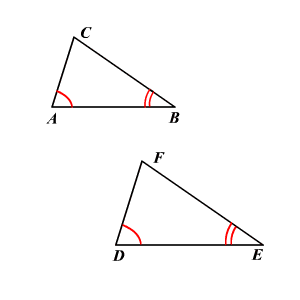# Angle-Angle Similarity

If two angles of a triangle are congruent to two angles of another triangle, then the two triangles are similar .If ,

then the triangles are similar.